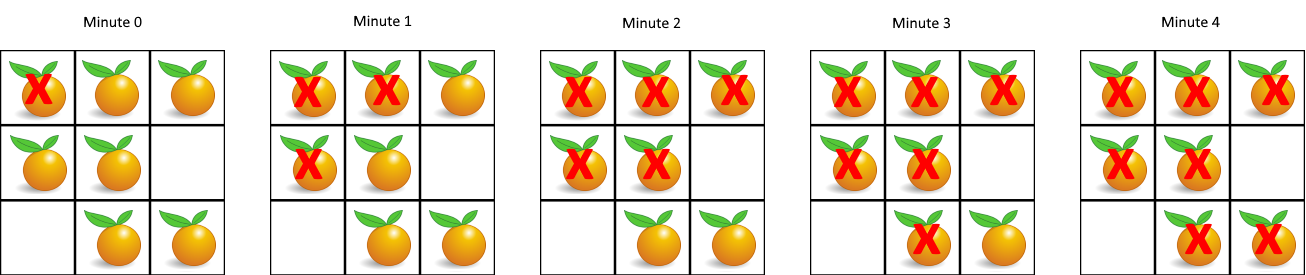## Algorithm

Problem Name: 994. Rotting Oranges

You are given an `m x n` `grid` where each cell can have one of three values:

• `0` representing an empty cell,
• `1` representing a fresh orange, or
• `2` representing a rotten orange.

Every minute, any fresh orange that is 4-directionally adjacent to a rotten orange becomes rotten.

Return the minimum number of minutes that must elapse until no cell has a fresh orange. If this is impossible, return `-1`.

Example 1:```Input: grid = [[2,1,1],[1,1,0],[0,1,1]]
Output: 4
```

Example 2:

```Input: grid = [[2,1,1],[0,1,1],[1,0,1]]
Output: -1
Explanation: The orange in the bottom left corner (row 2, column 0) is never rotten, because rotting only happens 4-directionally.
```

Example 3:

```Input: grid = [[0,2]]
Output: 0
Explanation: Since there are already no fresh oranges at minute 0, the answer is just 0.
```

Constraints:

• `m == grid.length`
• `n == grid[i].length`
• `1 <= m, n <= 10`
• `grid[i][j]` is `0`, `1`, or `2`.

## Code Examples

### #1 Code Example with Java Programming

```Code - Java Programming```

``````
class Solution {
private final int[][] DIRS = {{1, 0}, {0, 1}, {-1, 0}, {0, -1}};

public int orangesRotting(int[][] grid) {
int nonRottenCount = 0;
int numRows = grid.length;
int numCols = grid.length;
for (int i = 0; i < numRows; i++) {
for (int j = 0; j < numCols; j++) {
if (grid[i][j] == 2) {
} else if (grid[i][j] == 1) {
nonRottenCount++;
}
}
}
int totalTime = 0;
while (!queue.isEmpty() && nonRottenCount > 0) {
int size = queue.size();
while (size-- > 0) {
int[] removed = queue.remove();
for (int[] dir : DIRS) {
int newX = removed + dir;
int newY = removed + dir;
if (newX >= 0 && newY >= 0 && newX < numRows && newY < numCols && grid[newX][newY] == 1) {
grid[newX][newY] = 2;
nonRottenCount--;
}
}
}
totalTime++;
}
return nonRottenCount == 0 ? totalTime : -1;
}
}
``````
Copy The Code &

Input

cmd
grid = [[2,1,1],[1,1,0],[0,1,1]]

Output

cmd
4

### #2 Code Example with Javascript Programming

```Code - Javascript Programming```

``````
const orangesRotting = function(grid) {
const m = grid.length, n = grid.length
const dirs = [[-1, 0], [1, 0], [0, -1], [0, 1]]
const visited = new Set()
let q = []
let num = 0
for(let i = 0; i < m; i++) {
for(let j = 0; j < n; j++) {
if(grid[i][j] === 2) q.push([i, j]), visited.add(`\${i},\${j}`)
if(grid[i][j] !== 0) num++
}
}
let res = 0
while(q.length) {
const size = q.length
const tmp = []
for(let i = 0; i < size; i++) {
const [x, y] = q[i]
for(let [dx, dy] of dirs) {
const nx = x + dx, ny = y + dy
if(nx >= 0 && nx < m && ny >= 0 && ny < n && grid[nx][ny] === 1 && !visited.has(`\${nx},\${ny}`)) {
tmp.push([nx, ny])
}
}
}
q = tmp
if(q.length) res++
}
return visited.size === num ? res : -1
};
``````
Copy The Code &

Input

cmd
grid = [[2,1,1],[1,1,0],[0,1,1]]

Output

cmd
4

### #3 Code Example with Python Programming

```Code - Python Programming```

``````
class Solution:
def orangesRotting(self, grid: List[List[int]]) -> int:
bfs, t, m, n = [(i, j) for i, row in enumerate(grid) for j, val in enumerate(row) if val == 2], 0, len(grid), len(grid)
while bfs:
new = []
for i, j in bfs:
for x, y in ((i - 1, j), (i + 1, j), (i, j - 1), (i, j + 1)):
if 0 <= x < m and 0 <= y < n and grid[x][y] == 1:
grid[x][y] = 2
new.append((x, y))
bfs = new
t += bool(bfs)
return t if all(val != 1 for row in grid for val in row) else -1
``````
Copy The Code &

Input

cmd
grid = [[2,1,1],[0,1,1],[1,0,1]]

Output

cmd
-1

### #4 Code Example with C# Programming

```Code - C# Programming```

``````
using System.Collections.Generic;

namespace LeetCode
{
public class _0994_RottingOranges
{
public int OrangesRotting(int[][] grid)
{
var rows = grid.Length;
var cols = grid.Length;

var queue = new Queue<(int r, int c)>();
var freshCount = 0;
for (int i = 0; i < rows; i++)
for (int j = 0; j < cols; j++)
{
if (grid[i][j] == 2) queue.Enqueue((i, j));
else if (grid[i][j] == 1) freshCount++;
}

if (freshCount == 0) return 0;

var directions = new (int d_row, int d_col) { (0, 1), (1, 0), (0, -1), (-1, 0) };
var time = 0;
while (queue.Count > 0)
{
time++;
var size = queue.Count;
for (int i = 0; i < size; i++)
{
(int row, int col) = queue.Dequeue();
foreach ((int d_row, int d_col) in directions)
{
var neighborRow = row + d_row;
var neighborCol = col + d_col;
if (neighborRow >= 0 && neighborRow < rows &&
neighborCol >= 0 && neighborCol < cols)
{
if (grid[neighborRow][neighborCol] == 1)
{
grid[neighborRow][neighborCol] = 2;
freshCount--;
queue.Enqueue((neighborRow, neighborCol));
}
}
}
}

if (freshCount == 0) break;
}

return freshCount == 0 ? time : -1;
}
}
}
``````
Copy The Code &

Input

cmd
grid = [[2,1,1],[0,1,1],[1,0,1]]

Output

cmd
-1